# Olympiad Test: Patterns - 2

## 20 Questions MCQ Test National Cyber Olympiad Class 5 | Olympiad Test: Patterns - 2

Description
Attempt Olympiad Test: Patterns - 2 | 20 questions in 40 minutes | Mock test for Class 5 preparation | Free important questions MCQ to study National Cyber Olympiad Class 5 for Class 5 Exam | Download free PDF with solutions
QUESTION: 1

### Find the missing element: 3F, 6G, 11I, 18L, ?

Solution:

The letters move 1, 2, 3, ... steps forward. The numbers increase by + 3, + 5, + 7, + 9....

QUESTION: 2

### Find the missing element: D-4, F-6, H-8, J-10, ?, ?

Solution:

The letters are alternate and the numbers indicate their position in the alphabet from the beginning.

QUESTION: 3

### Find the missing element: D-4, F-6, H-8, J-10, ?, ?

Solution:

The letters are alternate and the numbers indicate their position in the alphabet from the beginning.

QUESTION: 4

Find the missing element: 2A11, 4D13, 12G17, ?

Solution:

The first numbers in the terms follow the sequence × 2, × 3, × 4. The letter moves three steps forward. The last numbers follow the sequence + 2, + 4, + 6.

QUESTION: 5

Find the missing element: C4X, F9U, 116R, ?

Solution:

The first letter moves two steps forward. The last letter moves one step backward. The number series runs as follows:
1 × 1 + 1 = 2
2 × 2 + 2 = 6
6 × 3 + 3 = 21
21 × 4 + 4 = 88.

QUESTION: 6

Find the missing element: B, D, F, I, L, P, ?

Solution:

The letters move 2, 2, 3, 3, 4, 4, ... steps forward.

QUESTION: 7

Find the missing element: BAZ, DCY, FEX, ?

Solution:

The first letter moves two steps forward. The second letter moves 2 steps forward. The third letter moves one step backward.

QUESTION: 8

Find the missing element: FLP, INS, LPV, ?

Solution:

The first letter moves three steps forward. The second letter moves two steps forward. The third letter moves three steps forward.

QUESTION: 9

Find the missing element: M, N, O, L, R, I, V, ?

Solution:

The series consists of two groups (M, O, R, V) and (N, L, I, ?). The letters in the first group move 2, 3, 4, ... steps forward. The letters in the second group move 2, 3, 4, ... steps backward.

QUESTION: 10

Find the missing element: BAS, ?, DCQ, DDP, FEO

Solution:

The first letter repeats once and subsequently moves one step forward. The second letter moves one step forward. The third letter moves one step backward.

QUESTION: 11

Find the missing element: DEF, HIJ, MNO, ?

Solution:

The letters are in alphabetical order. The gap between the last letter and the first letter of the next term keeps increasing in 1, 2, 3, ... steps.

QUESTION: 12

Find the missing element: Z, U, Q, ?, L

Solution:

The letters move 5, 4, 3, ... steps backward.

QUESTION: 13

Find the missing element: BYW, DUX, FQY, ?

Solution:

The first letter moves two steps forward. The second letter moves 4 steps backward. The third letter moves one step forward.

QUESTION: 14

Find the missing element: DFE, JIH, MLN, ?, VUT

Solution:

Each term consists of consecutive letters slightly jumbled up. There is a gap of three letters between the first letter and the last letter of the next term.

QUESTION: 15

Find the missing element: EJO, TYD, INS, XCH ?

Solution:

There is a gap of four letters between the first and second, the second and third letters. There is a gap of four letters between the last letter and the first letter of the next term in alphanumerical series.

QUESTION: 16

Identify the figure that completes the pattern.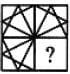Solution: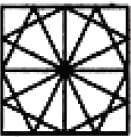QUESTION: 17

Identify the figure that completes the pattern.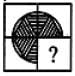Solution: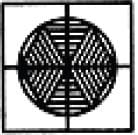QUESTION: 18

Identify the figure that completes the pattern.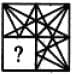Solution: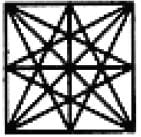QUESTION: 19

Identify the figure that completes the pattern.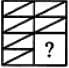Solution: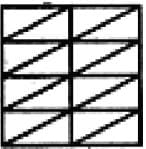QUESTION: 20

Identify the figure that completes the pattern.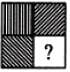Solution: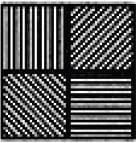Use Code STAYHOME200 and get INR 200 additional OFF Use Coupon Code